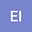Some new soliton solutions of time fractional resonant Davey-Stewartson equations
•## Abstract

In this study, via the Bernoulli sub-equation, the analytical traveling wave solution of the (2+1)-dimensional resonant Davey-Stewartson system is investigated. In the beginning, Based on the Riemann-Liouville fractional derivative, the time-fractional imaginary (2+1)-dimensional resonant Davey-Stewatson equation by using travelling wave is changed into a nonlinear differential system. The homogeneous balance method between the highest power terms and the highest derivative of the ordinary differential equation is authorized on the resultant outcome equation, and finally, the ordinary differential equations are solved to obtain some new exact solutions. Different cases, as well as different values of physical constants to investigate the optical soliton solutions of the resulting system, are used. The outcomes results of this study are shown in 3D dimensions graphically via Wolfram Mathematica Package.

#### Peer review status:UNDER REVIEW

06 Jul 2021Submitted to Mathematical Methods in the Applied Sciences
28 Jul 2021Assigned to Editor
28 Jul 2021Submission Checks Completed
24 Aug 2021Reviewer(s) Assigned